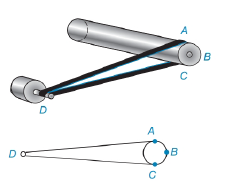Chapter 6.3, Problem 33EElementary Geometry For College St...

7th Edition
Alexander + 2 others
ISBN: 9781337614085

Solutions

Chapter
SectionElementary Geometry For College St...

7th Edition
Alexander + 2 others
ISBN: 9781337614085
Textbook Problem

The cylindrical brush on a vacuum cleaner is powered by an electric motor. In the figure, the drive shaft is at point D. if m A C ^ = 160 ° , find the measure of the angle formed by the drive belt at point D; that is find m ∠ DTo determine

To find:

To find mD.

Explanation

Given that, mAC^=160°.

From the diagrammatic representation,

Let D is vertex outside the circle therefore,

mD=mABC^-mAC^2

Since mABC^=360°-mAC^

Substitute mABC^=360°-mAC^ in the above equation to get he following,

mD=360°-mAC^-mAC^2

mD=

Still sussing out bartleby?

Check out a sample textbook solution.

See a sample solution

The Solution to Your Study Problems

Bartleby provides explanations to thousands of textbook problems written by our experts, many with advanced degrees!

Get Started

Find more solutions based on key concepts